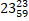# Quiz Discussion

The average of nine number is 50. The average of first five numbers is 54 and that of the last three numbers is 52. Then the sixth number is :

Course Name: Quantitative Aptitude

• 1] 30
• 2] 34
• 3] 24
• 4] 44
##### Solution
No Solution Present Yet

#### Top 5 Similar Quiz - Based On AI&ML

Quiz Recommendation System API Link - https://fresherbell-quiz-api.herokuapp.com/fresherbell_quiz_api

# Quiz
1
Discuss

Distance between two stations A and B is 778km. A train covers the journey from A to B at 84 km per hour and returns back to A with a uniform speed of 56 km per hour. Find the average speed of train during the whole journey.

• 1]

60 km/hr

• 2]

30.5 km/hr

• 3]

57 km/hr

• 4]

67.2 km/hr

##### Solution
2
Discuss

The average of six numbers is x and the average of three of these is y. If the average of the remaining three is z, then-

• 1] x = y + z
• 2] 2x = y + z
• 3] x = 2y + 2z
• 4] None of these
##### Solution
3
Discuss

A train covers the first 16 km at a speed of 20 km per hour another 20 km at 40 km per hour and the last 10 km at 15 km per hour. Find the average speed for the entire journey.

• 1]

24 km

• 2]

26 km

• 3]

21 km

• 4]km

##### Solution
4
Discuss

There are five boxes in cargo hold. The weight of the first box is 200 kg and the weight of the second box is 20% higher than the weight of the third box, whose weight is 25% higher than the first box's weight. The fourth box at 350 kg is 30% lighter than the fifth box. Find the difference in the average weight of the four heaviest boxes and the four lightest boxes.

• 1] 51.5 kg
• 2] 75 kg
• 3] 37.5 kg
• 4] 112.5 kg
• 5] None of these
##### Solution
5
Discuss

The average of 20 numbers is zero. Of them, at the most, how many may be greater than zero ?

• 1]

0

• 2]

-1

• 3]

1

• 4]

None Of These

##### Solution
6
Discuss

The average of 25 results is 18. The average of first 12 of those is 14 and the average of last 12 is 17. What is the 13th result?

• 1] 74
• 2] 75
• 3] 69
• 4] 78
##### Solution
7
Discuss

Ten years ago, the ages of the members of a joint family of eight people added up to 231 years. Three years later, one member died at the age of 60 years and a child was born during the same year. After another three years, one more member died, again at 60, and a child was born during the same year. The current average of this eight-member joint family is nearest to-

• 1] 21 years
• 2] 22 years
• 3] 23 years
• 4] 24 years
• 5] 25 years
##### Solution
8
Discuss

The average age of three boys is 15 years. If their ages are in ratio 3 : 5 : 7, the age of the youngest boy is

• 1] 21 years
• 2] 18 years
• 3] 15 years
• 4] 9 years
• 5] 12 years
##### Solution
9
Discuss

There are two sections A and B of a class, consisting of 36 and 44 students' respectively. If the average weight of section A is 40kg and that of section B is 35kg, find the average weight of the whole class.

• 1] 30 kg
• 2] 35 kg
• 3] 42.5 kg
• 4] 37.25 kg
##### Solution
10
Discuss

The average age of 30 students is 9 years. If the age of their teacher is included, the average age becomes 10 years. The age of the teacher (in years) is :

• 1] 27
• 2] 31
• 3] 35
• 4] 40
# Quiz